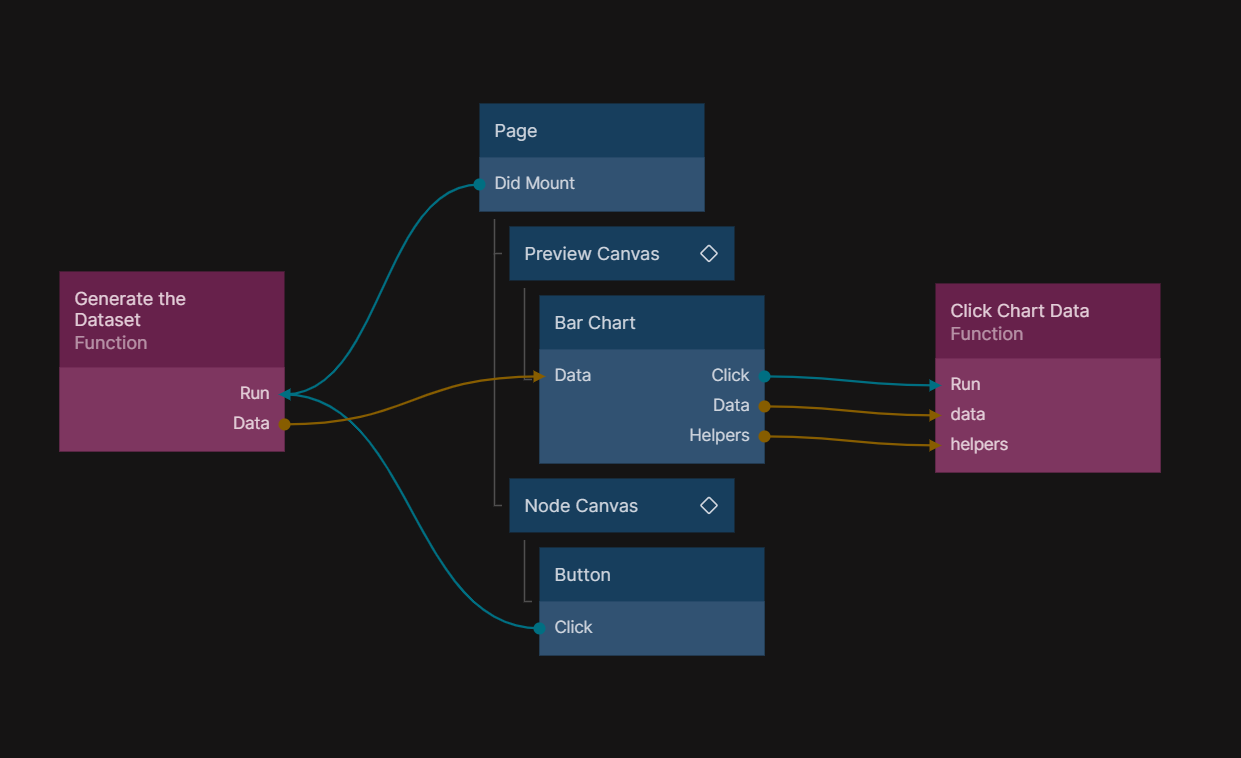# Interactions

This guide is based on the documentation from Chart.js, which can be found here.

## Converting Events to Data Values​

A common occurrence is taking an event, such as a click, and finding the data coordinates on the chart where the event occurred. Chart.js provides helpers that make this a straightforward process.In this screenshot, we connect the "Click", "Data" and "Helpers" outputs to a function node to be able to process the chart data.

With this code in the Function node, we can start by getting the values on the X and Y axis.

``const data = Inputs.data;const helpers = Inputs.helpers;const canvasPosition = helpers.getRelativePosition(data, data.chart);// Substitute the appropriate scale IDsconst dataX = data.chart.scales.x.getValueForPixel(canvasPosition.x);const dataY = data.chart.scales.y.getValueForPixel(canvasPosition.y);``

Now with `dataX` and `dataY` we know what values the user clicked on.

To continue from here, you can follow Chart.js guides.

There are for examples getPixelForValue, that returns the location of the given data point.

### Before Event​

Make all the connections under the "Before Event" category and helpers from the "Click Event" category, and this function will be triggered on any of the before events.

``const chart = Inputs.chart;const args = Inputs.args;const helpers = Inputs.helpers;const canvasPosition = helpers.getRelativePosition(args.event, chart);// Substitute the appropriate scale IDsconst dataX = chart.scales.x.getValueForPixel(canvasPosition.x);const dataY = chart.scales.y.getValueForPixel(canvasPosition.y);console.log({  canvasPosition,  dataX,  dataY,});``